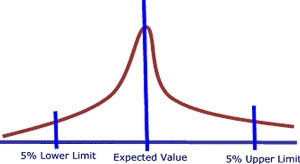# Uncertainty – lack of information

This entry is part 3 of 6 in the series Monte Carlo Simulation

Every item in a budget or a profit and loss account represents in reality a probability distribution. In this framework all items whether from the profit and loss account or from the balance sheet will have individual probability distributions. These distributions are generated by the combination of distributions from factors of production that define the item.

Variance will increase as we move down the items in the profit and loss account. The message is that even if there is a low variance in the input variables (sales, prices, costs etc.) metrics like NOPLAT, Free Cash Flow and Economic Profit will have a much higher variance.

The key issue is to identify the various items and establish the individual probability distribution. This can take place by using historical data, interviewing experts or comparing data from other relevant sources. There are three questions we need to answer to define the proportions of the uncertainty:

• What is the expected value?
• What is the lowest likely value?
• What is the highest likely value?

When we have decided the limits where we with 95% probability estimate the result to be within we then decide what kind of probability distribution is relevant for the item. There are several to choose among, but we will emphasize three types here.

1. The Normal Distribution
2. The Skewed Distribution
3. The Triangular Distribution

The Normal Distribution is being used when we have situations where there is a likeliness for a symmetric result. It can be a good result but has the same probability of being bad.

The Skew Distribution is being used when it can occur situations where we are lucky and experience more sales than we expected and vice versa we can experience situations where expenditure is less than expected.

The Triangular Distribution is being used when we are planning investments. This is due to the fact that we tend to know fairly well what we expect to pay and we know we will not get merchandise for free and there is a limit for how much we are willing to pay.

When we have defined the limits for the uncertainty where we with 95% probability estimate the result to be within we can start to calculate the risk and prioritize the items that matters in terms of creating value or loss.Series Navigation<< The ChallengeRisk – Exposure to Gain and Loss >>